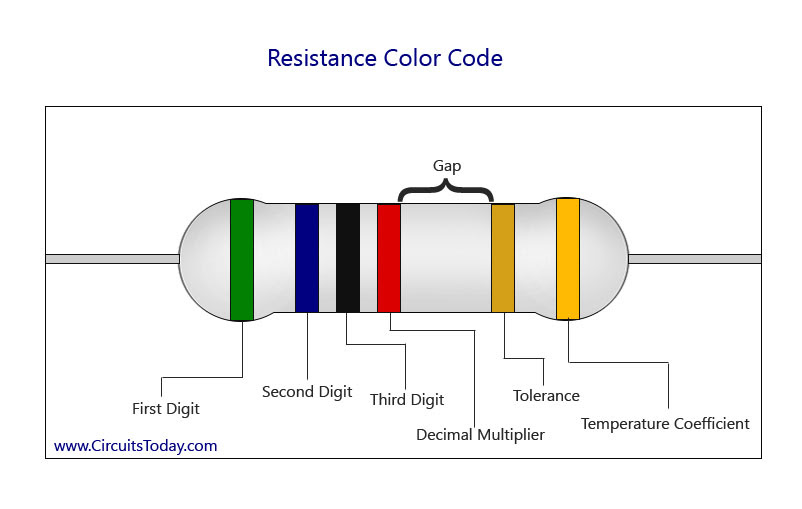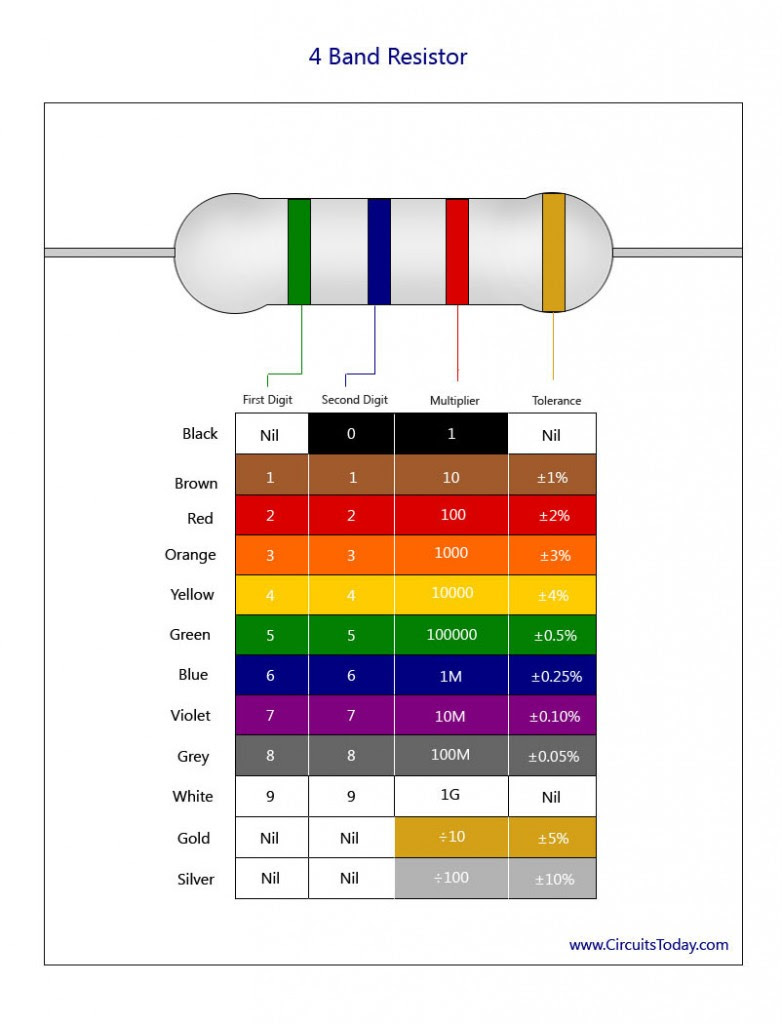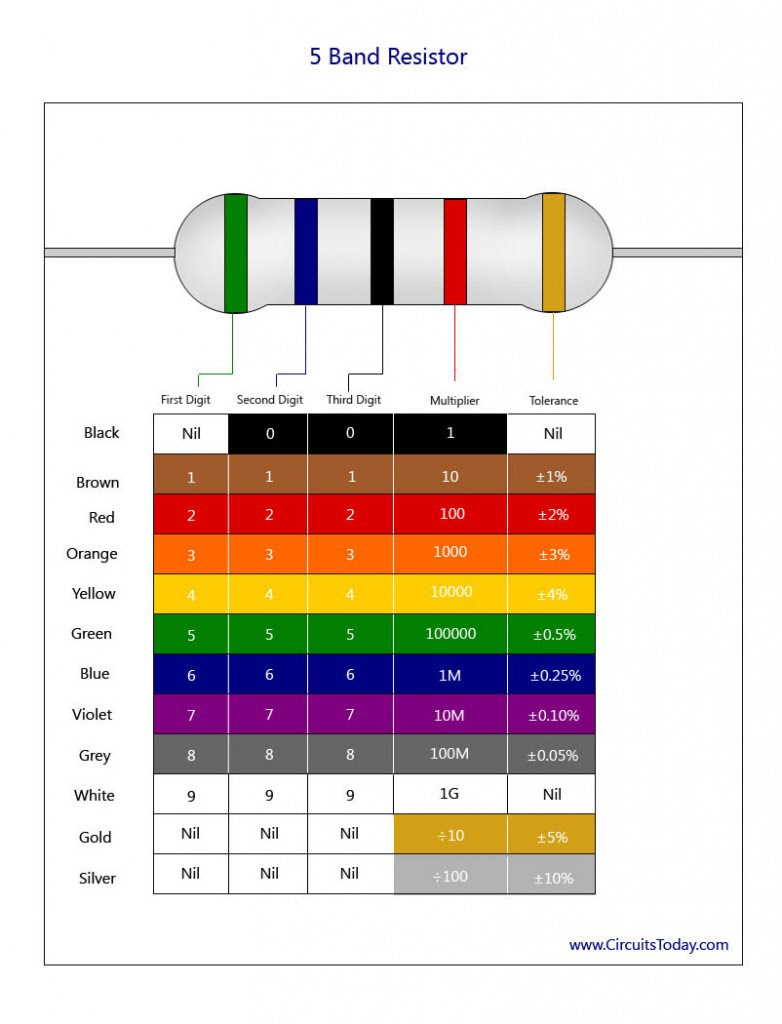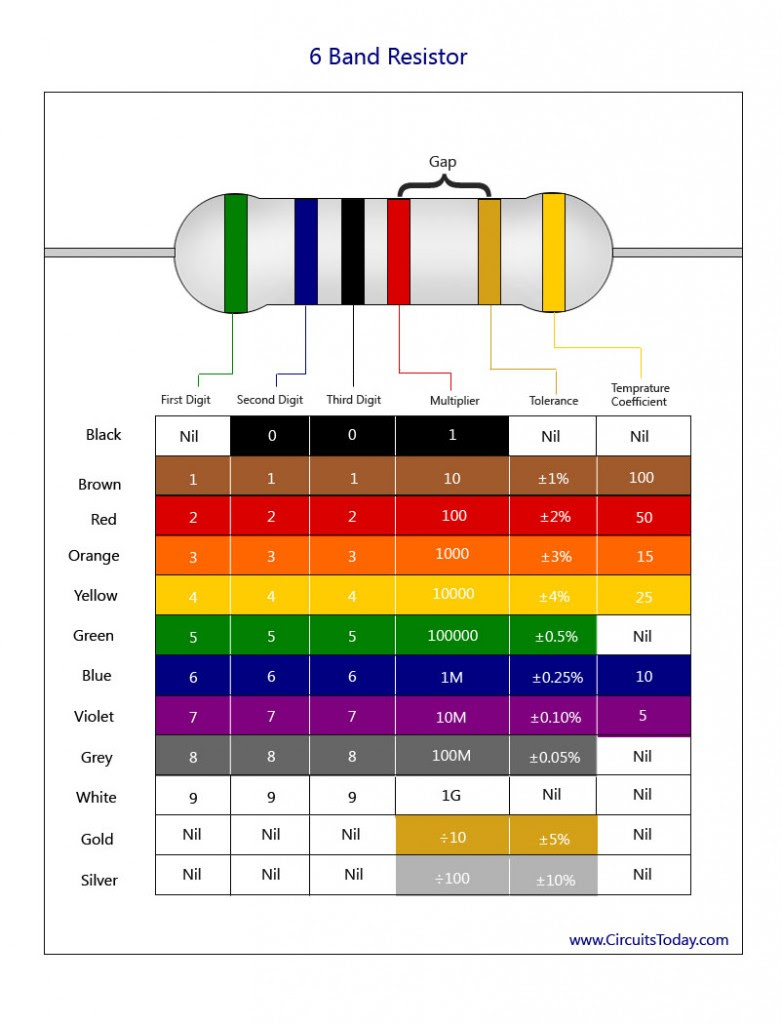## Audio

[Audio][slideshow]

“Color coding” is used in electronics to identify between different components. In the case of resistors, color coding is used to identify a specific resistance value, for example a 100 ohms resistor or a 1 kilo ohms resistor with 5% tolerance. Electronic components like resistors are very small in size and its difficult to print its value directly on to the component surface. Hence a standard was formed in 1920 by then Radio Manufacturers Association (now part of EIA – Electronic Industries Alliance) to identify values and ratings of electronic components by printing color codes on them. Color coding technique makes it easy to print values (based on color codes) on small components, such as resistors and facilitates cost effective manufacturing.

This technique of “color coding” has 2 disadvantages. The first one appeals to general users where it becomes difficult to distinguish between colors (for example “Red” and “Brown” ) when the component is over heated. But this is not a major concern as the exact value can be easily identified using a multimeter (in case of confusion). The next drawback is for a specific group of people – color blind people can not identify the device using color codes. However they too can depend on multimeter to identify resistance values.

### How to Identify Resistor Color Code

The figure below shows the layout of the bands, the multiplier and the tolerance value of a resistor. For a 6-band resistor, an additional temperature coefficient band is provided.The gap between the multiplier and the tolerance specifies the left and right side of the resistor. So here are the key points;

4 band resistor – has 3 color bands on left side and one color band on right side. First two bands represent significant digits, the 3rd band represents multiplier and the fourth band on right side represents tolerance.

5 band resistor – has 4 color bands on left side and one color band on right side. Here the first 3 color bands represent significant digits, fourth one represent multiplier and the 5th one on right side represents tolerance.

6 band resistor – has 4 color bands on left side and 2 color bands on right side. Here the first 3 color bands represents significant digits, fourth one represents multiplier, 5th one represents tolerance and the 6th one represents temperature coefficient of the resistor.

In a 4-band resistor, the first two bands represent the first two digits of the resistor. The multiplier band indicates the value that is to be multiplied with the first two digits. The tolerance band after the multiplier band indicates the range of accuracy of the resistor. It is represented in units of percentage. In case of 5 band resistor, the decimal multiplier will be assigned to the fourth band and tolerance value will be assigned to the fifth band. Finally in case of a 6 band resistor, the last band (i.e 6th band) represents temperature coefficient. .The sixth temperature coefficient band increases the precision of the resistance value. Temperature coefficient tells us the behavior of resistor under different heating conditions (means the variation in resistance values under normal conditions and over heated conditions)It is defined in units of ppm/K.

### Resistor Color Code Chart

We have presented 3 charts below – which are exactly resistor color code charts for 4 band, 5 band and 6 band resistors respectively.

#### 4-Band Resistor Color Code Identification

Let us consider the color code for the resistor with the bands BROWN-BLACK-RED-GOLD. Brown corresponds to the value ‘1’ in the color chart. Black represents ‘0’ and Red represents the multiplier ‘100’. Thus the value of the resistance to the corresponding color code is 10*100 = 1000 ohms or 1 kilo ohm with the tolerance band being Gold which represents a tolerance of +/- 5%. Thus the actual value of the 1 kilo ohm can be between 950 ohms and 1050 ohms.Band 1 – First digit value of resistor

Band 2 – Second digit value of resistor

Band 3 – Decimal Multiplier

Band 4 – Tolerance Value

#### 5-Band Resistor Color Code Identification

Let us consider the color code for the resistor with the bands YELLOW-VIOLET-BLACK-BROWN-GREY. Yellow corresponds to the value ‘4’ in the color chart. Violet represents ‘7’ and Black represents the value ‘0’. Brown represents the multiplier ‘10’. Thus the value of the resistance to the corresponding color code is 470*10 = 4700 ohms or 4.7 kilo ohm with the tolerance band being Grey which represents a tolerance of +/- 0.05%.Band 1 – First digit value of resistor

Band 2 – Second digit value of resistor

Band 3 – Third digit value of resistor

Band 4 – Decimal Multiplier

Band 5 – Tolerance Value

#### 6-Band Resistor Color Code Identification

Here also, if we can consider the same color code used for the 5-band, with an additional temperature coefficient band with a blue color. This shows that the resistor has a value of 4.7 kilo ohms, with tolerance +/- 0.05% and with a temperature coefficient of 10 ppm/K.Band 1 – First digit value of resistor

Band 2 – Second digit value of resistor

Band 3 – Third digit value of resistor

Band 4 – Decimal Multiplier

Band 5 – Tolerance Value

Band 6 – Temperature Coefficient

Note:- Nowadays with advances in printing technology, its possible to print numeric values on small components as well. If you look at an SMD resistor (surface mount), you will see the resistance value printed directly on the surface of the resistor.

[Lights][grids]